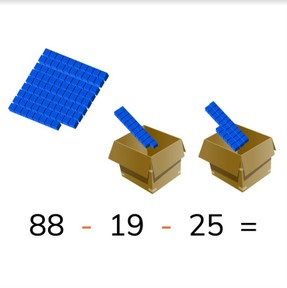Subtraction to 100 with three or more numbers

# Subtraction to 100 with three or more numbers8,000 schools use Gynzy92,000 teachers use Gynzy1,600,000 students use Gynzy

## General

Students learn to subtract numbers to 100 with three numbers.

2.NBT.B.5
2.OA.A.1

## Relevance

It is important to be able to subtract numbers to 100 with multiple numbers so you can determine how much you have left when multiple numbers are taken away. An example would be if you hand out water bottles at school on different days and you want to know how many you have left after three days.

## Introduction

Practice two subtraction problems with visual support on the interactive whiteboard to get students thinking about subtraction.

## Development

First discuss with students the importance of being able to subtract numbers to 100 with three numbers. Then the learning goal is presented in three ways, with visual support, in the abstract, and as story problems. Use the blue menu in the bottom right to select the method which best suits your classroom needs. In the abstract it is also taught that you can swap the order of the second and third subtrahends. And in the story problems you can choose to click the speaker button and the story problem will be read aloud.

Check that students are able to subtract problems with numbers to 100 with three or more numbers by asking the following questions:
- Why is it useful to be able to subtract more than two numbers at a time?
- What do you do first when subtracting multiple numbers, for example 65 - 43 - 15?
- Why can it be useful to swap the order of the second and third subtrahends?

## Guided practice

Students are given subtraction problems with visual support, as abstract problems, and are given story problems to solve.

## Closing

Practice two more subtraction problems as a class as well as having the students solve two more story problems individually or in pairs. Then close the lesson with a game. Have the students stand in a circle. One student must stand in the middle of the circle and say a subtraction problem with three numbers. They then close their eyes, hold out their arm straight ahead of them and spin three times in a circle. The student they are pointing at when they stop must say the difference. If they have the correct answer, they may take place in the middle of the circle.

## Teaching tips

Students who have difficulty with this learning goal can be supported by the use of MAB blocks or other manipulatives.

## Instruction materials

Optional: MAB blocks or other manipulatives

### The online teaching platform for interactive whiteboards and displays in schools

• Save time building lessons

• Manage the classroom more efficiently

• Increase student engagement